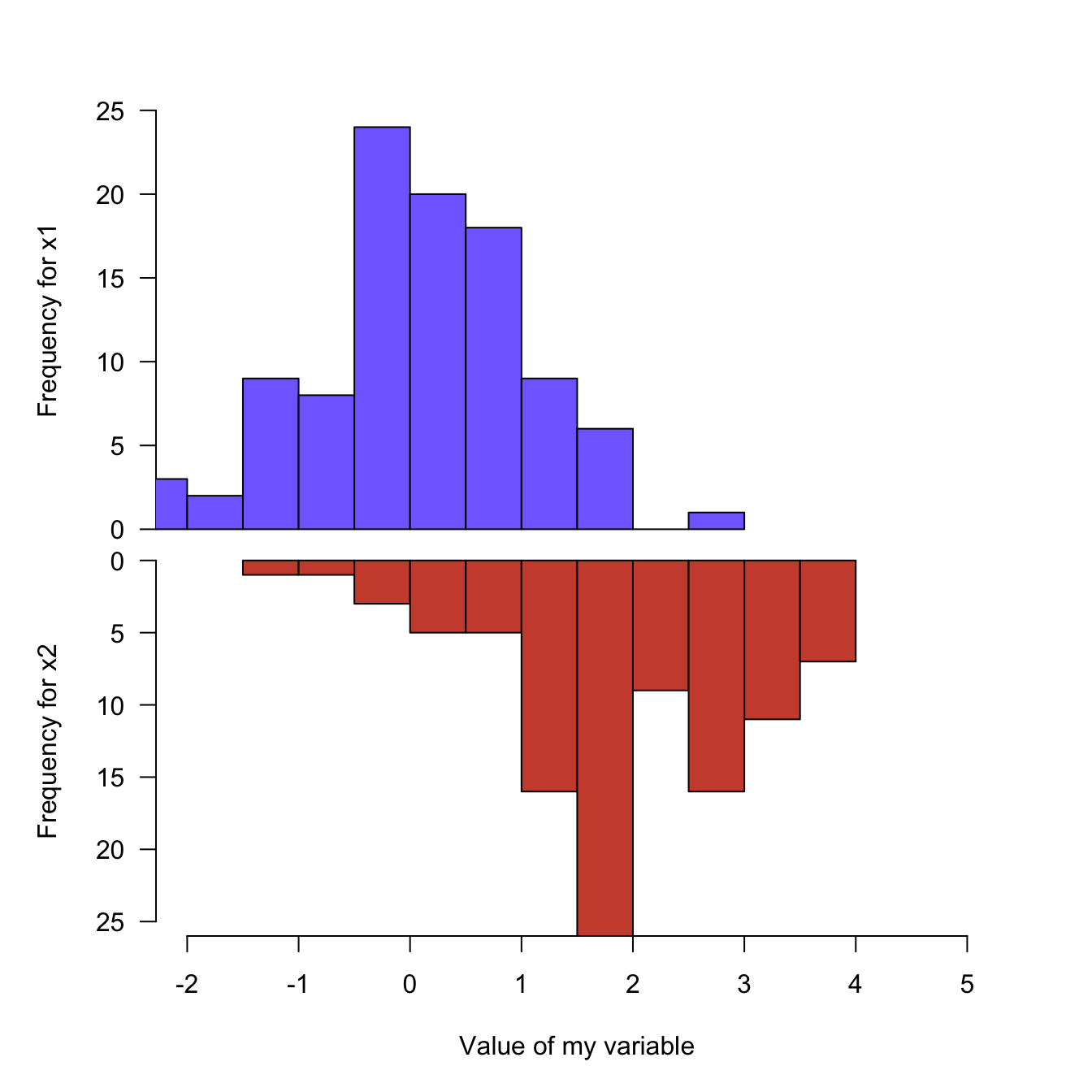# Mirrored histogram in base R

A histogram displays the distribution of a numeric variable. This posts explains how to build a mirrored histogram in base R, without any package. It allows to compare the distribution of 2 variables

The mirrored histogram allows to compare the distribution of 2 variables.

First split the screen with the `par(mfrow())` command. The top histogram needs a `xaxt="n"` statement to discard its X axis. For the second one, inverse the values of the `ylim` argument to flip it upside down. Use the `margin` command to adjust the position of the 2 charts.``````#Create Data
x1 = rnorm(100)
x2 = rnorm(100)+rep(2,100)
par(mfrow=c(2,1))

#Make the plot
par(mar=c(0,5,3,3))
hist(x1 , main="" , xlim=c(-2,5), ylab="Frequency for x1", xlab="", ylim=c(0,25) , xaxt="n", las=1 , col="slateblue1", breaks=10)
par(mar=c(5,5,0,3))
hist(x2 , main="" , xlim=c(-2,5), ylab="Frequency for x2", xlab="Value of my variable", ylim=c(25,0) , las=1 , col="tomato3"  , breaks=10)``````

Related chart types

## Contact

This document is a work by Yan Holtz. Any feedback is highly encouraged. You can fill an issue on Github, drop me a message on Twitter, or send an email pasting yan.holtz.data with gmail.com.